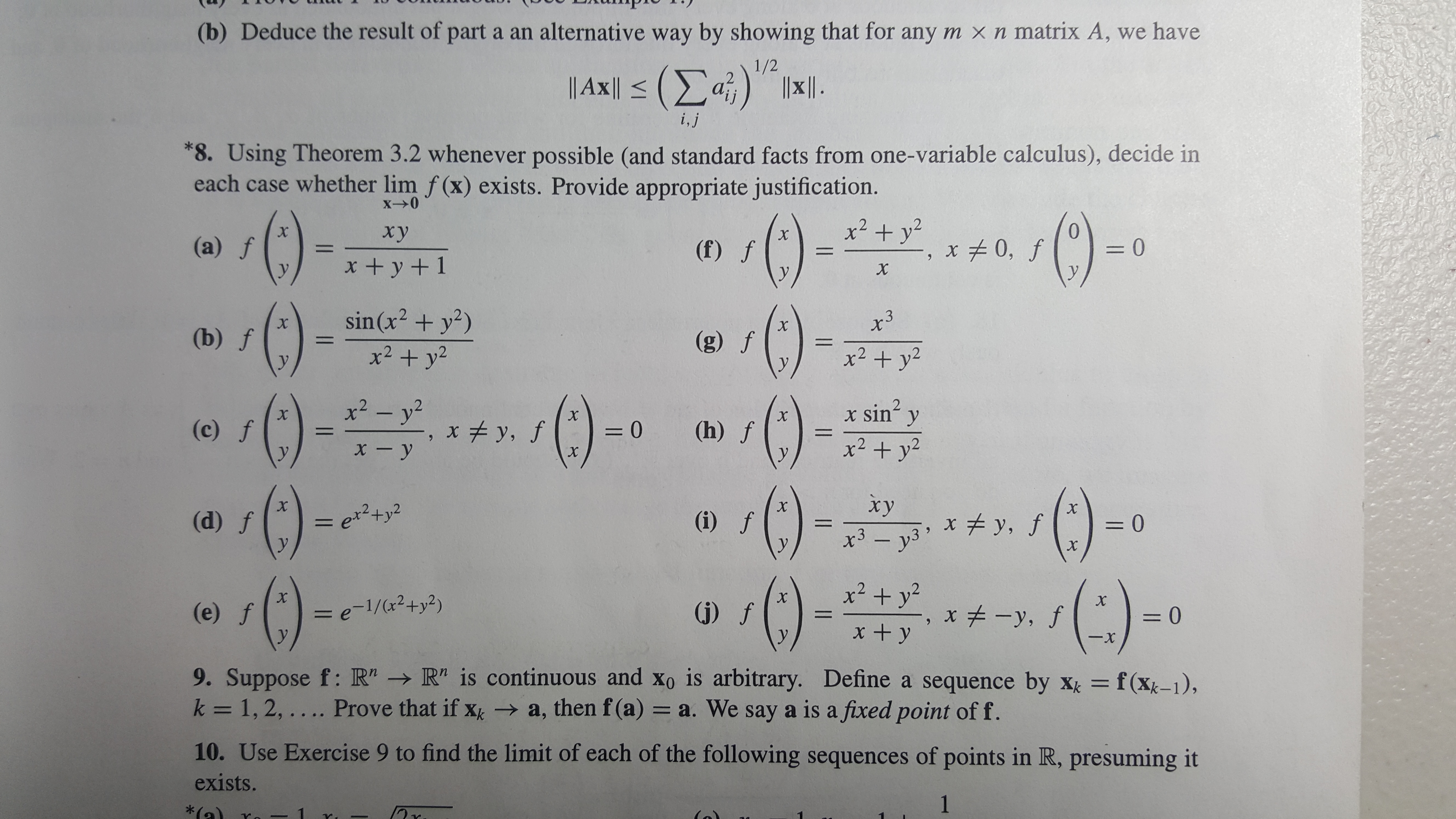# (b) Deduce the result of part a an alternative way by showing that for any m xn matrix A, we have 1/2 | Ax| <(Σα) i,j 8. Using Theorem 3.2 whenever possible (and standard facts from one-variable calculus), decide in each case whether lim f (x) exists. Provide appropriate justification. XO () () C) 0-0 x2 y X xy X (a) f (f) f = 0 X x +y+1 X sin (x2y2) x3 X (b) f (g) f x2y2 x2 - y2 sin2 x X Xx X y (c) f xy, f (h) f X 1 xy x2 y2 X xy X X (d) f exty2 (i) f = 0 1 X 3-y3 y, f Xr X ()- () x2 +y2 ()- X X (e) f = e-1/(r2+y2) (j) f x# -y, f = 0 y x + y 9. Suppose f: R" R" is continuous and xo is arbitrary. Define a sequence by xk f(x-1), k = 1, 2, .... Prove that if x a, then f(a) = a. We say a is a fixed point off. 10. Use Exercise 9 to find the limit of each of the following sequences of points in R, presuming it exists. 1

Questionhelp_outlineImage Transcriptionclose(b) Deduce the result of part a an alternative way by showing that for any m xn matrix A, we have 1/2 | Ax| <(Σα) i,j 8. Using Theorem 3.2 whenever possible (and standard facts from one-variable calculus), decide in each case whether lim f (x) exists. Provide appropriate justification. XO () () C) 0-0 x2 y X xy X (a) f (f) f = 0 X x +y+1 X sin (x2y2) x3 X (b) f (g) f x2y2 x2 - y2 sin2 x X Xx X y (c) f xy, f (h) f X 1 xy x2 y2 X xy X X (d) f exty2 (i) f = 0 1 X 3-y3 y, f Xr X ()- () x2 +y2 ()- X X (e) f = e-1/(r2+y2) (j) f x# -y, f = 0 y x + y 9. Suppose f: R" R" is continuous and xo is arbitrary. Define a sequence by xk f(x-1), k = 1, 2, .... Prove that if x a, then f(a) = a. We say a is a fixed point off. 10. Use Exercise 9 to find the limit of each of the following sequences of points in R, presuming it exists. 1 fullscreen

### Want to see this answer and more?

Experts are waiting 24/7 to provide step-by-step solutions in as fast as 30 minutes!*

*Response times vary by subject and question complexity. Median response time is 34 minutes and may be longer for new subjects.
Tagged in
MathCalculus

### Limits